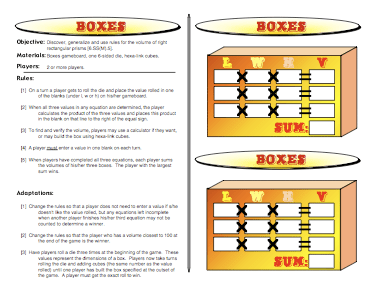# Boxes: Finding Volume GameThe Boxes Game helps kids tie together a formula for volume, with a 2-D representation of the rectangular prism, with an actual 3-D prism built with hexa-link cubes. This game offers students an opportunity to understand the concept of volume more fully.They’re actually taking the space that is created inside this prism and measuring it with cubes. When first using the formula for the volume of a rectangular prism, kids are usually somewhat confused about which dimension of the prism is the length, which is the width, and which is the height. This practice helps solidify how the dimensions are specified, but it also shows them that since multiplication is both commutative and associative, the order in which they multiply the dimensions will not impact their final answer for the volume.

It’s a good idea to get students into the habit of stating the measurement they are using. In other words, the volume should be described as inches or centimeters cubed (or to the third power). That idea, the one of a cubed measurement, needs to be built up gradually in the classroom as students are using linear measurement and then work up to area followed by volume.

But with all games there’s an element of chance, and the luck of the die will determine which player wins this game. Another fun adaptation of this game in a classroom setting is to split the room into teams. The teacher will roll the die and then the teams can quickly build the prisms and compute their volumes. Whoever finishes first with the correct volume answers as well as the sum of the volumes, wins.

Common Core Mathematical Standard
7.G Solve real-life and mathematical problems involving angle measure, area, surface area, and volume.

Common Core Standards for Mathematical Practice
4. Model with mathematics.